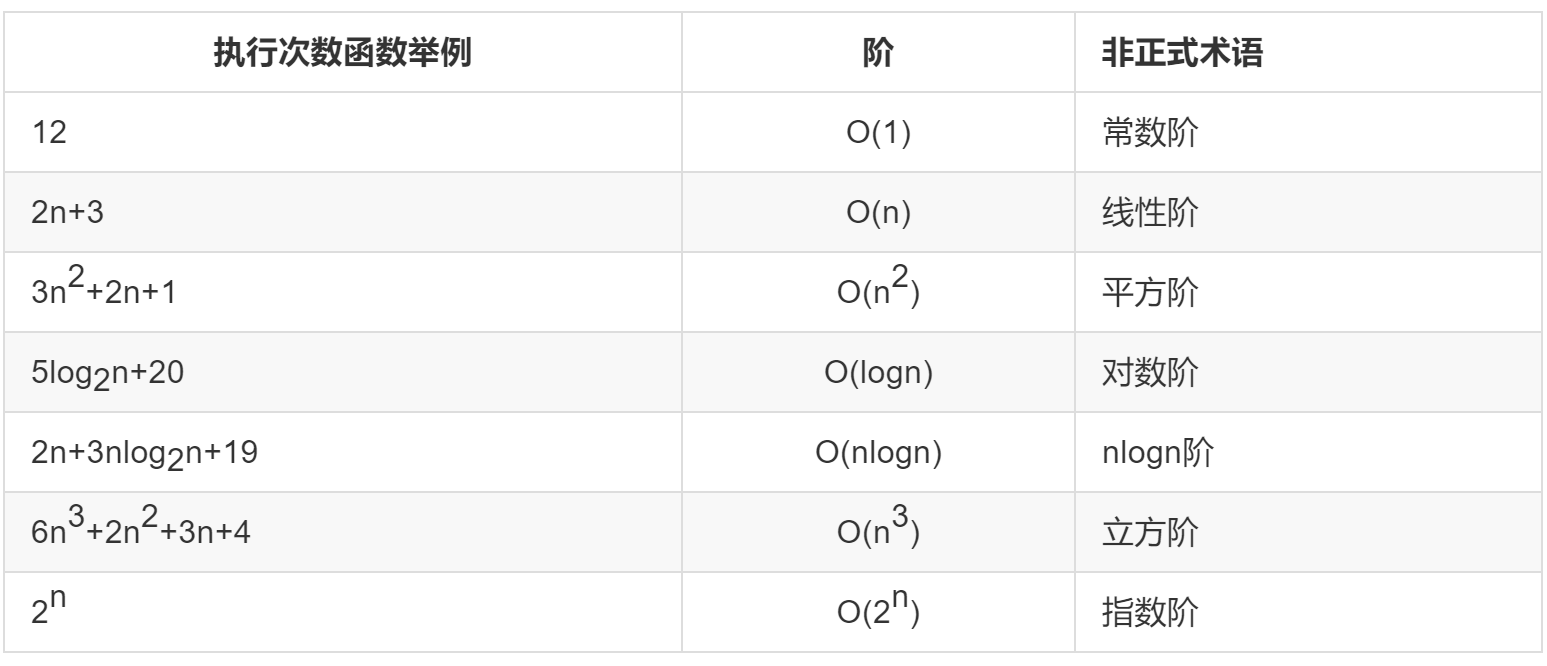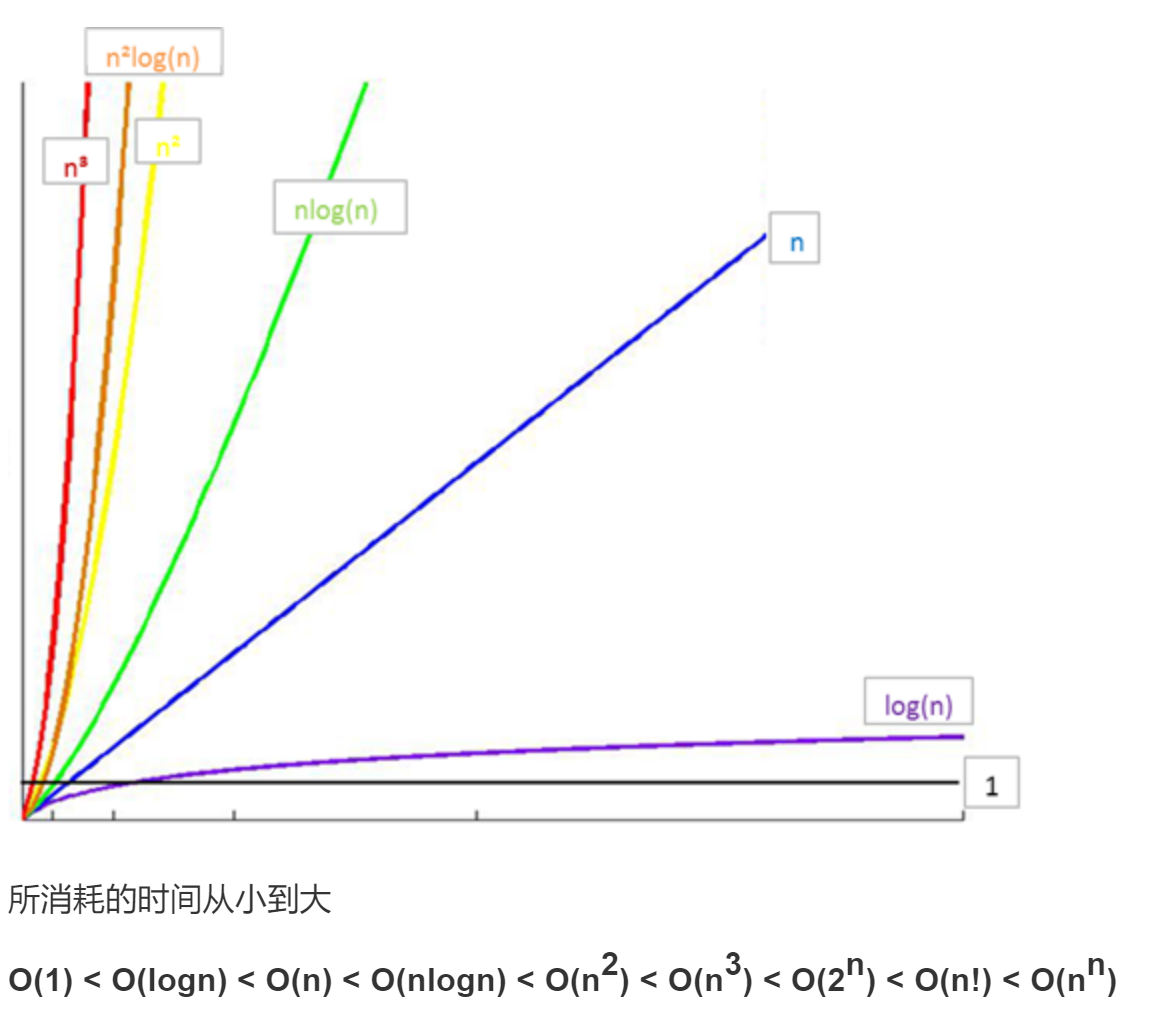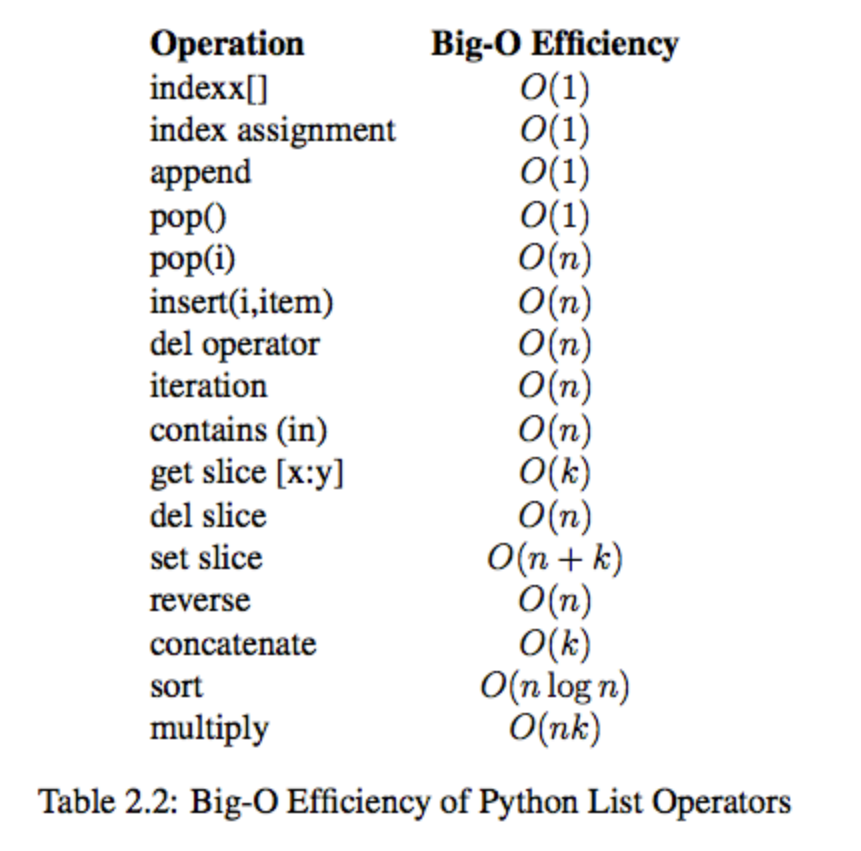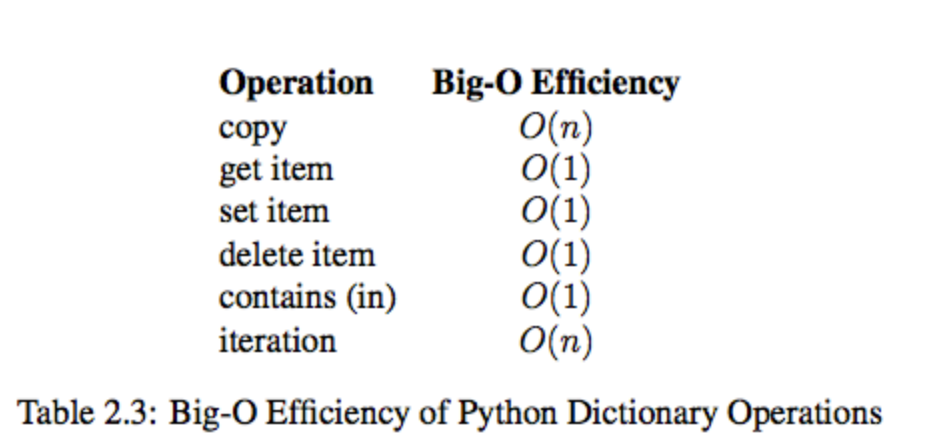# Python数据结构与算法（一） 时间复杂度 数据结构1173-杨同学

# 算法效率衡量

## 时间复杂度与“大O记法”

“大O记法”：对于单调的整数函数f，如果存在一个整数函数g和实常数c>0，使得对于充分大的n总有f(n)<=c*g(n)，就说函数g是f的一个渐近函数（忽略常数），记为f(n)=O(g(n))。也就是说，在趋向无穷的极限意义下，函数f的增长速度受到函数g的约束，亦即函数f与函数g的特征相似。

## 常见时间复杂度# Python内置类型性能分析

## timeit模块

timeit模块可以用来测试一小段Python代码的执行速度。

class timeit.Timer(stmt=‘pass’, setup=‘pass’, timer=)
Timer是测量小段代码执行速度的类。

stmt参数是要测试的代码语句（statment）；

setup参数是运行代码时需要的设置；

timer参数是一个定时器函数，与平台有关。

timeit.Timer.timeit(number=1000000)
Timer类中测试语句执行速度的对象方法。number参数是测试代码时的测试次数，默认为1000000次。方法返回执行代码的平均耗时，一个float类型的秒数。

### list的操作测试

def test1():
l = []
for i in range(1000):
l = l + [i]
def test2():
l = []
for i in range(1000):
l.append(i)
def test3():
l = [i for i in range(1000)]
def test4():
l = list(range(1000))

from timeit import Timer
#传入函数名的字符串，并非直接函数值
t1 = Timer("test1()", "from __main__ import test1")
print("concat ",t1.timeit(number=1000), "seconds")
t2 = Timer("test2()", "from __main__ import test2")
print("append ",t2.timeit(number=1000), "seconds")
t3 = Timer("test3()", "from __main__ import test3")
print("comprehension ",t3.timeit(number=1000), "seconds")
t4 = Timer("test4()", "from __main__ import test4")
print("list range ",t4.timeit(number=1000), "seconds")

# ('concat ', 1.7890608310699463, 'seconds')
# ('append ', 0.13796091079711914, 'seconds')
# ('comprehension ', 0.05671119689941406, 'seconds')
# ('list range ', 0.014147043228149414, 'seconds')


pop操作测试

x = range(2000000)
pop_zero = Timer("x.pop(0)","from __main__ import x")
print("pop_zero ",pop_zero.timeit(number=1000), "seconds")
x = range(2000000)
pop_end = Timer("x.pop()","from __main__ import x")
print("pop_end ",pop_end.timeit(number=1000), "seconds")

# ('pop_zero ', 1.9101738929748535, 'seconds')
# ('pop_end ', 0.00023603439331054688, 'seconds')


pop最后一个元素的效率远远高于pop第一个元素

## list内置操作的时间复杂度## dict内置操作的时间复杂度# 数据结构

## 概念

Python给我们提供了很多现成的数据结构类型，这些系统自己定义好的，不需要我们自己去定义的数据结构叫做Python的内置数据结构，比如列表、元组、字典。而有些数据组织方式，Python系统里面没有直接定义，需要我们自己去定义实现这些数据的组织方式，这些数据组织方式称之为Python的扩展数据结构，比如栈，队列等。

## 抽象数据类型(Abstract Data Type)

class Stus(object):
def pop
def sort
def modify


### 评论 抢沙发### 觉得文章有用就打赏一下文章作者

#### 支付宝扫一扫打赏#### 微信扫一扫打赏Vieu3.3主题Q Q 登 录